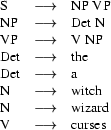## 6.1 Review of DCGs

Let's review some basic facts about DCGs. What follows is not intended as a complete introduction (for that, see last semester's notes, or any decent Prolog textbook). It's simply meant to remind you of some basic aspects of DCG notation, and how DCGs are used.

### 6.1.1 DCGs are a natural notation for context free grammars

Suppose we are working with a context free grammar, for example, this:We can immediately turn this into the following DCG:

s --> np,vp.np --> det,n.vp --> v,np.det --> [the].det --> [a].n --> [witch].n --> [wizard].v --> [curses].

The link between the two formalisms is obvious. About the only thing you have to remember is that in DCG notation, lexical items (that is, the words) have to be written as elements of a one-element list.

What's not quite so obvious is the way DCGs are used. For example, to see whether A witch curses the wizard'' is accepted by the grammar we need to pose the following query:

s([a,witch,curses,the,wizard],[]).

That is, we have to give two arguments, one of which is the empty list. Similarly, to see if the witch'' is a noun phrase in this grammar, we would pose the query

np([the,witch],[]).

Recall that DCGs can be used to generate (a very useful property). For example, to see all the sentences generated by our little grammar, we would give the following query:

s(X,[]).

To see all the noun phrases produced by the grammar we would pose the query:

np(X,[]).

### 6.1.2 DCGs are really syntactic sugar for difference lists

Why the two arguments when we make queries? Because DCGs are really syntactic sugar'' for something else. They are a nice user friendly notation for grammars written in terms of difference lists. For example, when the above grammar is given to Prolog, the Prolog interpreter will immediately translate the first rule into something like this:

s(A,B) :-    np(A,C),    vp(C,B).

On the other hand, the lexical rule for the'' will translate into something like this

det([the|W],W).

This is not the place to go into how this works (actually, by this stage, this should all be familiar) but I will make two remarks. First, the difference list representation of grammars, while a bit complicated at first sight, is a very efficient way of representing grammars. In particualr, the use of difference lists allows us to avoid using the append3/ predicate, thus DCGs are a useful way of writing even quite large grammars. Second, notice that the translated clauses all have two arguments --- which explains why the above queries needed two arguments.

Summing up: DCG notation is a natural notation that lets us write context free grammars in a natural way. DCGs translate into a difference list representation that allows far more efficient processing than a naive single-list representation that relies on using append3/.

### 6.1.3 DCGs give us a natural notation for features

But we can do more with DCGs. For a start, we are able to add extra arguments to our DCG rules. And this lets us do some linguistically useful things --- and in particular it lets us use features.

Suppose we wanted to deal with sentences like She shoots him'', and He shoots her''. What should we do? Well, obviously we should add rules saying that he'', she'', him'', and her'' are pronouns:

pro --> [he].pro --> [she].pro --> [him].pro --> [her].

Furthermore, we should add a rule saying that noun phrases can be pronouns:

np --> pro.

This new DCG (kind of) works. For example:

s([she,shoots,him],[]).yes

But there's obvious problems. The DCG will also accept a lot of sentences that are clearly wrong, such as A witch curses she'', Her curses a wizard'', and Her shoots she''.

Here's a bad way of repairing the problem: add more rules.\ For example, we might rewrite the DCG as follows:

s --> np_subject,vp.np_subject --> det,n.np_object  --> det,n.np_subject --> pro_subject.np_object  --> pro_object.vp --> v,np_object. vp --> v.det --> [the].det --> [a].n --> [witch].n --> [wizard].pro_subject --> [he].pro_subject --> [she].pro_object --> [him].pro_object --> [her].v --> [curses].

This is awful. Basically, we've had to make a big change to the grammar to cope with a very small set of facts. After all, let's face it: I'' and me'' are pretty much the same --- they just differ with respect to their case property and the way they sound. By marking information with features, we can do a much neater job:

s --> np(subject),vp.np(_) --> det,n.np(X) --> pro(X).vp --> v,np(object).vp --> v. det --> [the].det --> [a].n --> [witch].n --> [wizard].v --> [curse].pro(subject) --> [he].pro(subject) --> [she].pro(object) --> [him].pro(object) --> [her].

The extra argument --- the feature --- is simply passed up the tree by ordinary unification. And, depending on whether it can correctly unify or not, this feature controls the facts of English case neatly and simply.

Summing up: features let us get rid of lots of unnecessary rules in a natural way. And DCGs enable us to implement rules with feature information in a natural way.

One last remark. Note that extra arguments, really are just plain old Prolog arguments. For example

s --> np(subject),vp.

translates into something like.

s(A,B) :-    np(subject,A,C),    vp(C,B).

Patrick Blackburn and Kristina Striegnitz
Version 1.2.4 (20020829)